Create a new printableAnalyze Data and Graphs
Math Worksheets

Sample - Click above to make a new math worksheet (PDF).
 Name _____________________________Date ___________________
Ordered Pairs
Draw the ordered points on the grid. Label each point.

 1 * This is a pre-made sheet.Use the link at the top of the page for a printable page.
2.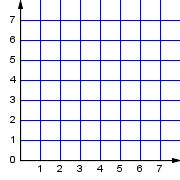C  (3, 0)R  (0, 0)G  (0, 6)T  (7, 6)Z  (0, 7)Y  (2, 6)
3.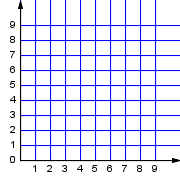X  (4, 6)Z  (4, 5)P  (9, 6)A  (8, 8)F  (3, 3)Q  (0, 9)
4.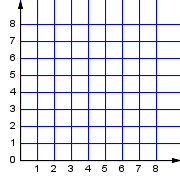F  (0, 6)K  (5, 6)Q  (4, 3)B  (3, 0)S  (7, 2)D  (4, 1)
5.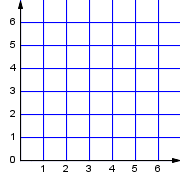N  (6, 0)H  (0, 0)V  (6, 3)R  (2, 5)Q  (2, 1)T  (5, 1)
6.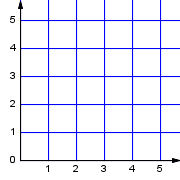Y  (3, 4)Q  (5, 3)M  (4, 0)B  (5, 2)C  (3, 2)T  (0, 3)